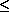Instant recognition of half integrality and 2-approximation

## Abstract

We define a class of integer programs with constraints that involve up to three variables each. A generic constraint in such integer program is of the form ax + byz + c, where the variable z appears only in that constraint. For such binary integer programs it is possible to derive half integral superoptimal solutions in polynomial time. The scheme is also applicable with few modifications to nonbinary integer problems. For some of these problems it is possible to round the half integral solution to a 2-approximate solution. This extends the class of integer programs with at most two variables per constraint that were analyzed in HMNT93. Interesting problems for which we can get superoptimal half integral solutions include the sparsest cut problem, and minimum weight edge deletion to obtain a bipartite graph. Problems for which we can get 2-approximations include minimum satisfiability, scheduling with precedence constraints, minimum weight node deletion to obtain a complete bipartite graph, several boolean functions on two variables (generalized 2SAT) and the feasible cut problems. The technique described here is used also to derive a 2-approximation algorithm for a complement of the maximum clique problem. In addition we cast a large class of problems as minimum satisfiability problems and a recognition procedure for determining when such problems are 2-approximable. The approximation algorithms here provide an improvement in running time and range of applicability compared to existing 2-approximations. Furthermore, we conclude that problems in the framework are MAX SNP-hard and at least as hard to approximate as vertex cover.
Problems that are amenable to the analysis provided here are easily recognized. The analysis itself is entirely technical and involves manipulating the constraints and transforming them to a totally unimodular system while losing (for binary problems) no more than a factor of 2 in the integrality.